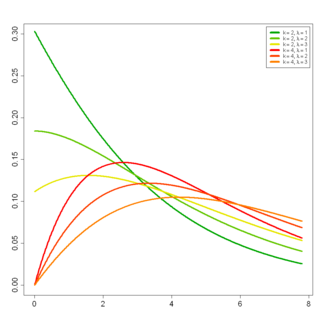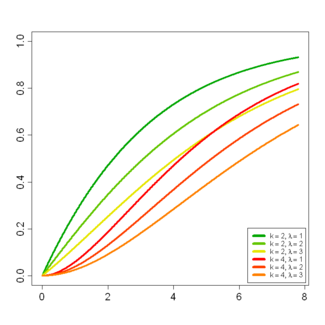# Noncentral chi-squared distribution

﻿
Noncentral chi-squared distribution
parameters: Probability density functionCumulative distribution function$k > 0\,$ degrees of freedom$\lambda > 0\,$ non-centrality parameter$x \in [0; +\infty)\,$$\frac{1}{2}e^{-(x+\lambda)/2}\left (\frac{x}{\lambda} \right)^{k/4-1/2} I_{k/2-1}(\sqrt{\lambda x})$$1 - Q_{\frac{k}{2}} \left( \sqrt{\lambda}, \sqrt{x} \right)$ with Marcum Q-function QM(a,b)$k+\lambda\,$$2(k+2\lambda)\,$$\frac{2^{3/2}(k+3\lambda)}{(k+2\lambda)^{3/2}}$$\frac{12(k+4\lambda)}{(k+2\lambda)^2}$$\frac{\exp\left(\frac{ \lambda t}{1-2t }\right)}{(1-2 t)^{k/2}}$ for 2t < 1$\frac{\exp\left(\frac{i\lambda t}{1-2it}\right)}{(1-2it)^{k/2}}$

In probability theory and statistics, the noncentral chi-squared or noncentral χ2 distribution is a generalization of the chi-squared distribution. This distribution often arises in the power analysis of statistical tests in which the null distribution is (perhaps asymptotically) a chi-squared distribution; important examples of such tests are the likelihood ratio tests.

## Background

Let Xi be k independent, normally distributed random variables with means μi and variances$\sigma_i^2$. Then the random variable$\sum_{i=1}^k \left(\frac{X_i}{\sigma_i}\right)^2$

is distributed according to the noncentral chi-squared distribution. It has two parameters: k which specifies the number of degrees of freedom (i.e. the number of Xi), and λ which is related to the mean of the random variables Xi by:$\lambda=\sum_{i=1}^k \left(\frac{\mu_i}{\sigma_i}\right)^2.$

λ is sometime called the noncentrality parameter. Note that some references define λ in other ways, such as half of the above sum, or its square root.

This distribution arises in multivariate statistics as a derivative of the multivariate normal distribution. While the central chi-squared distribution is the squared norm of a random vector with N(0k,Ik) distribution (i.e., the squared distance from the origin of a point taken at random from that distribution), the non-central χ2 is the squared norm of a random vector with N(μ,Ik) distribution. Here 0k is a zero vector of length k, μ = (μ1,...,μk) and Ik is the identity matrix of size k.

## Properties

### Probability density function

The probability density function is given by$f_X(x; k,\lambda) = \sum_{i=0}^\infty \frac{e^{-\lambda/2} (\lambda/2)^i}{i!} f_{Y_{k+2i}}(x),$

where Yq is distributed as chi-squared with q degrees of freedom.

From this representation, the noncentral chi-squared distribution is seen to be a Poisson-weighted mixture of central chi-squared distributions. Suppose that a random variable J has a Poisson distribution with mean λ / 2, and the conditional distribution of Z given J = i is chi-squared with k+2i degrees of freedom. Then the unconditional distribution of Z is non-central chi-squared with k degrees of freedom, and non-centrality parameter λ.

Alternatively, the pdf can be written as$f_X(x;k,\lambda)=\frac{1}{2} e^{-(x+\lambda)/2} \left (\frac{x}{\lambda}\right)^{k/4-1/2} I_{k/2-1}(\sqrt{\lambda x})$

where Iν(z) is a modified Bessel function of the first kind given by$I_a(y) = (y/2)^a \sum_{j=0}^\infty \frac{ (y^2/4)^j}{j! \Gamma(a+j+1)} .$

Using the relation between Bessel functions and hypergeometric functions, the pdf can also be written as:$f_X(x;k,\lambda)={{\rm e}^{-\lambda/2}} _0F_1(;k/2;\lambda x/4)\frac{1}{2^{k/2}\Gamma(k/2)} {\rm e}^{-x/2} x^{k/2-1}.$

Siegel (1979) discusses the case k=0 specifically (zero degrees of freedom), in which case the distribution has a discrete component at zero.

### Moment generating function

The moment generating function is given by$M(t;k,\lambda)=\frac{\exp\left(\frac{ \lambda t}{1-2t }\right)}{(1-2 t)^{k/2}}.$

The first few raw moments are:$\mu^'_1=k+\lambda$$\mu^'_2=(k+\lambda)^2 + 2(k + 2\lambda)$$\mu^'_3=(k+\lambda)^3 + 6(k+\lambda)(k+2\lambda)+8(k+3\lambda)$$\mu^'_4=(k+\lambda)^4+12(k+\lambda)^2(k+2\lambda)+4(11k^2+44k\lambda+36\lambda^2)+48(k+4\lambda)$

The first few central moments are:$\mu_2=2(k+2\lambda)\,$$\mu_3=8(k+3\lambda)\,$$\mu_4=12(k+2\lambda)^2+48(k+4\lambda)\,$

The nth cumulant is$K_n=2^{n-1}(n-1)!(k+n\lambda).\,$

Hence$\mu^'_n = 2^{n-1}(n-1)!(k+n\lambda)+\sum_{j=1}^{n-1} \frac{(n-1)!2^{j-1}}{(n-j)!}(k+j\lambda )\mu^'_{n-j}.$

### Cumulative distribution function

Again using the relation between the central and noncentral chi-squared distributions, the cumulative distribution function (cdf) can be written as$P(x; k, \lambda ) = e^{-\lambda/2}\; \sum_{j=0}^\infty \frac{(\lambda/2)^j}{j!} Q(x; k+2j)$

where$Q(x; k)\,$ is the cumulative distribution function of the central chi-squared distribution which is given by$Q(x;k)=\frac{\gamma(k/2,x/2)}{\Gamma(k/2)}\,$
and where$\gamma(k,z)\,$ is the lower incomplete Gamma function.

The Marcum Q-function QM(a,b)can also be used to represent the cdf.$P(x; k, \lambda) = 1 - Q_{\frac{k}{2}} \left( \sqrt{\lambda}, \sqrt{x} \right)$

#### Approximation

Sankaran  discusses a number of closed form approximations for the cumulative distribution function. In an earlier paper, he derived and states the following approximation:$P(x; k, \lambda ) \approx \Phi \lbrace \frac{(\frac{x} {k + \lambda}) ^ h - (1 + h p (h - 1 - 0.5 (2 - h) m p))} {h \sqrt{ 2p} (1 + 0.5 m p)} \rbrace$

where$\Phi \lbrace \cdot \rbrace \,$ denotes the cumulative distribution function of the standard normal distribution;$h = 1 - \frac{2}{3} \frac{(k+ \lambda) (k+ 3 \lambda)}{(k+ 2 \lambda) ^ 2} \, ;$$p = \frac{k+ 2 \lambda}{(k+ \lambda) ^ 2} ;$$m = (h - 1) (1 - 3 h) \, .$

This and other approximations are discussed in a later text book.

To approximate the Chi-squared distribution, the non-centrality parameter,$\lambda\,$, is set to zero.

For a given probability, the formula is easily inverted to provide the corresponding approximation for$x\,$.

## Derivation of the pdf

The derivation of the probability density function is most easily done by performing the following steps:

1. First, assume without loss of generality that$\sigma_1=\ldots=\sigma_k=1$. Then the joint distribution of$X_1,\ldots,X_k$ is spherically symmetric, up to a location shift.
2. The spherical symmetry then implies that the distribution of$X=X_1^2+\ldots+X_k^2$ depends on the means only through the squared length,$\lambda=\mu_1^2+\ldots+\mu_k^2$. Without loss of generality, we can therefore take$\mu_1=\sqrt{\lambda}$ and$\mu_2=\dots=\mu_k=0$.
3. Now derive the density of$X=X_1^2$ (i.e. k=1 case). Simple transformation of random variables shows that :\begin{align}f_X(x,1,\lambda) &= \frac{1}{2\sqrt{x}}\left( \phi(\sqrt{x}-\sqrt{\lambda}) + \phi(\sqrt{x}+\sqrt{\lambda}) \right )\\ &= \frac{1}{\sqrt{2\pi x}} e^{-(x+\lambda)/2} \cosh(\sqrt{\lambda x}),\\ \end{align}
where$\phi(\cdot)$ is the standard normal density.
4. Expand the cosh term in a Taylor series. This gives the Poisson-weighted mixture representation of the density, still for k=1. The indices on the chi-squared random variables in the series above are 1+2i in this case.
5. Finally, for the general case. We've assumed, without loss of generality, that$X_2,\ldots,X_k$ are standard normal, and so$X_2^2+\ldots+X_k^2$ has a central chi-squared distribution with (k-1) degrees of freedom, independent of$X_1^2$. Using the poisson-weighted mixture representation for$X_1^2$, and the fact that the sum of chi-squared random variables is also chi-squared, completes the result. The indices in the series are (1+2i)+(k-1) = k+2i as required.

## Related distributions

• If V is chi-squared distributed$V \sim \chi_k^2$ then V is also non-central chi-squared distributed:$V \sim {\chi'}^2_k(0)$
• If$V_1 \sim {\chi'}_{k_1}^2(\lambda)$ and$V_2 \sim {\chi'}_{k_2}^2(0)$ and V1 is independent of V2 then a noncentral F-distributed variable is developed as$\frac{V_1/k_1}{V_2/k_2} \sim F'_{k_1,k_2}(\lambda)$
• If$J \sim Poisson(\frac{\lambda}{2})$, then$\chi_{k+2J}^2 \sim {\chi'}_k^2(\lambda)$
• Normal approximation: if$V \sim {\chi'}^2_k(\lambda)$, then$\frac{V-(k+\lambda)}{\sqrt{2(k+2\lambda)}}\to N(0,1)$ in distribution as either$k\to\infty$ or$\lambda\to\infty$.

### Transformations

Sankaran (1963) discusses the transformations of the form z = [(Xb) / (k + λ)]1 / 2. He analyzes the expansions of the cumulants of z up to the term O((k + λ) − 4) and shows that the following choices of b produce reasonable results:

• b = (k − 1) / 2 makes the second cumulant of z approximately independent of λ
• b = (k − 1) / 3 makes the third cumulant of z approximately independent of λ
• b = (k − 1) / 4 makes the fourth cumulant of z approximately independent of λ

Also, a simpler transformation z1 = (X − (k − 1) / 2)1 / 2 can be used as a variance stabilizing transformation that produces a random variable with mean (λ + (k − 1) / 2)1 / 2 and variance O((k + λ) − 2).

Usability of these transformations may be hampered by the need to take the square roots of negative numbers.

Various chi and chi-squared distributions
Name Statistic
chi-squared distribution$\sum_1^k \left(\frac{X_i-\mu_i}{\sigma_i}\right)^2$
noncentral chi-squared distribution$\sum_1^k \left(\frac{X_i}{\sigma_i}\right)^2$
chi distribution$\sqrt{\sum_1^k \left(\frac{X_i-\mu_i}{\sigma_i}\right)^2}$
noncentral chi distribution$\sqrt{\sum_1^k \left(\frac{X_i}{\sigma_i}\right)^2}$

Wikimedia Foundation. 2010.

### Look at other dictionaries:

• Noncentral chi-square distribution — Probability distribution name =Noncentral chi square type =density pdf cdf parameters =k > 0, degrees of freedom lambda > 0, non centrality parameter support =x in [0; +infty), pdf =frac{1}{2}e^{ (x+lambda)/2}left (frac{x}{lambda} ight)^{k/4 1/2} …   Wikipedia

• Chi-squared distribution — This article is about the mathematics of the chi squared distribution. For its uses in statistics, see chi squared test. For the music group, see Chi2 (band). Probability density function Cumulative distribution function …   Wikipedia

• Noncentral chi distribution — Noncentral chi parameters: degrees of freedom support: pdf …   Wikipedia

• Chi-square distribution — Probability distribution name =chi square type =density pdf cdf parameters =k > 0, degrees of freedom support =x in [0; +infty), pdf =frac{(1/2)^{k/2{Gamma(k/2)} x^{k/2 1} e^{ x/2}, cdf =frac{gamma(k/2,x/2)}{Gamma(k/2)}, mean =k, median… …   Wikipedia

• Chi distribution — chi Probability density function Cumulative distribution function parameters …   Wikipedia

• Noncentral F-distribution — In probability theory and statistics, the noncentral F distribution is a continuous probability distribution that is a generalization of the (ordinary) F distribution. It describes the distribution of the quotient (X/n1)/(Y/n2), where the… …   Wikipedia

• Noncentral t-distribution — Noncentral Student s t Probability density function parameters: degrees of freedom noncentrality parameter support …   Wikipedia

• Normal distribution — This article is about the univariate normal distribution. For normally distributed vectors, see Multivariate normal distribution. Probability density function The red line is the standard normal distribution Cumulative distribution function …   Wikipedia

• Probability distribution — This article is about probability distribution. For generalized functions in mathematical analysis, see Distribution (mathematics). For other uses, see Distribution (disambiguation). In probability theory, a probability mass, probability density …   Wikipedia

• Hypergeometric distribution — Hypergeometric parameters: support: pmf …   Wikipedia